Figures for "Impossible fractals"

Cameron Browne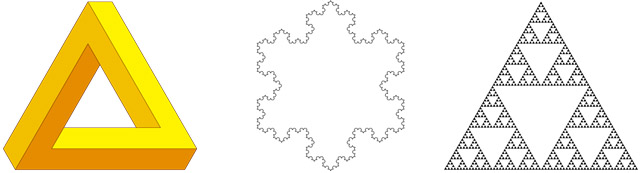Figure 1. The tri-bar, the Koch snowflake and the Sierpinski gasket.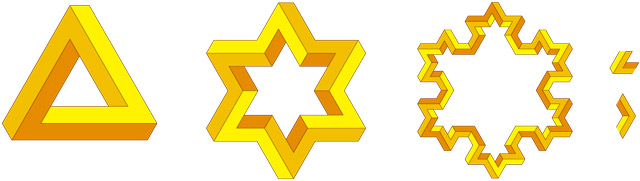Figure 2. Two iterations of an impossible snowflake (with acute and obtuse generators shown).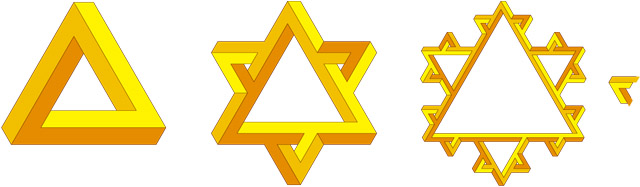Figure 3. An alternative snowflake design that emphasizes the perceptual effect (with generator shown).Figure 4. Impossible gaskets are more troublesome.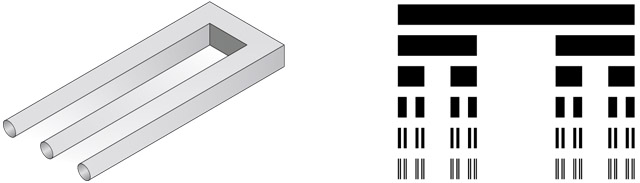Figure 5. The Devil's fork and the Cantor set.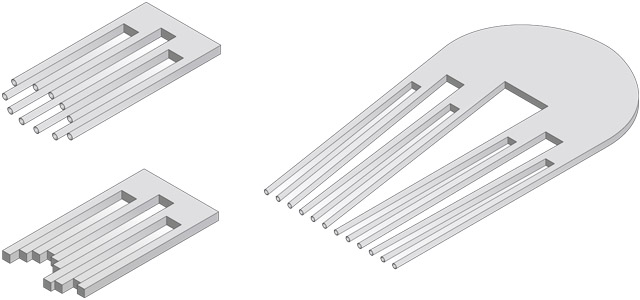Figure 6. The Devil's gatling gun (two levels), timber offcuts (two levels) and comb (three levels).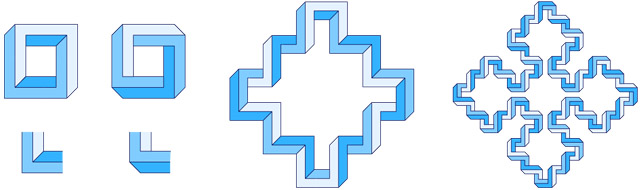Figure 7. Four-bar designs (with sharp and truncated generators) applied to the square Sierpinski curve,.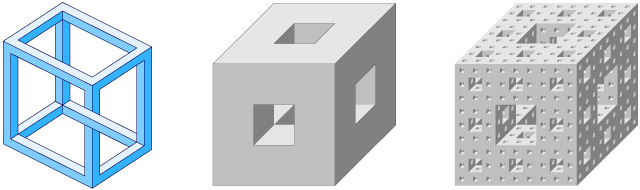Figure 8. An impossible multibar cube and the Menger sponge after one and three iterations.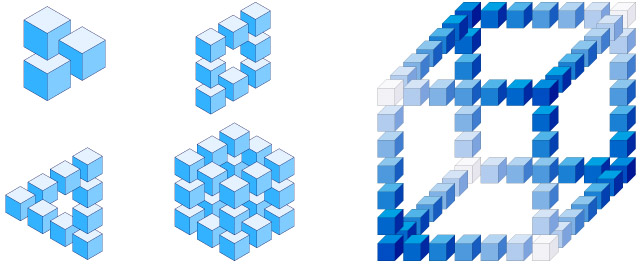Figure 9. Subcube designs and Buch's "Cube in blue".Figure 10. Ernst's "Nest of impossible cubes" and two variations.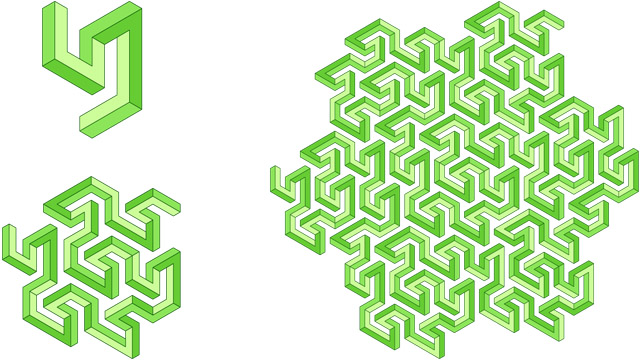Figure 11. An impossible multibar Peano-Gosper curve.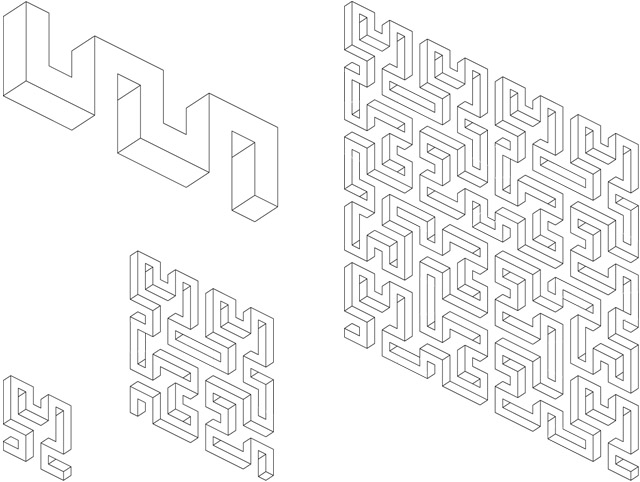Figure 12. Reutersvärd's "Meander" and a Hilbert meander.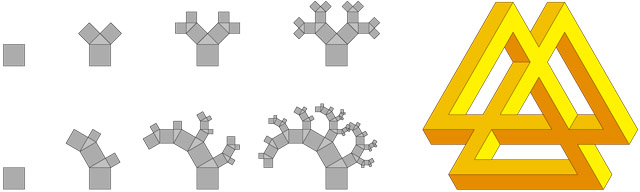Figure 13. 45° Pythagorean tree, balanced 30° Pythagorean tree and extended tri-bar.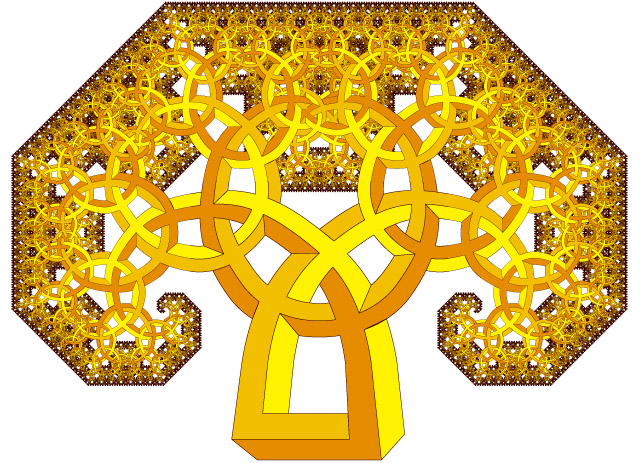Figure 14. An impossible mushroom (45° Pythagorean tree).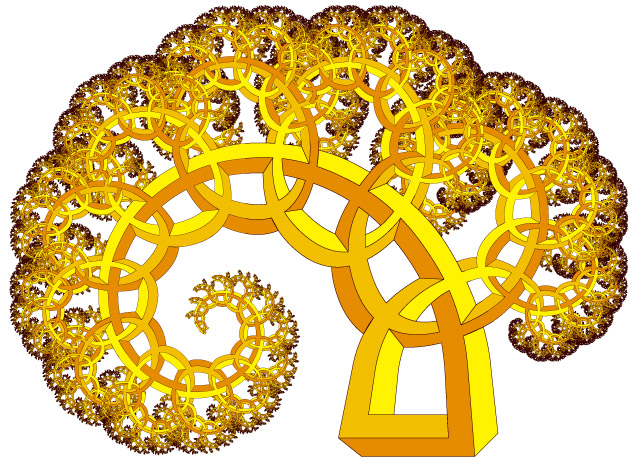Figure 15. An impossible fern (balanced 30° Pythagorean tree).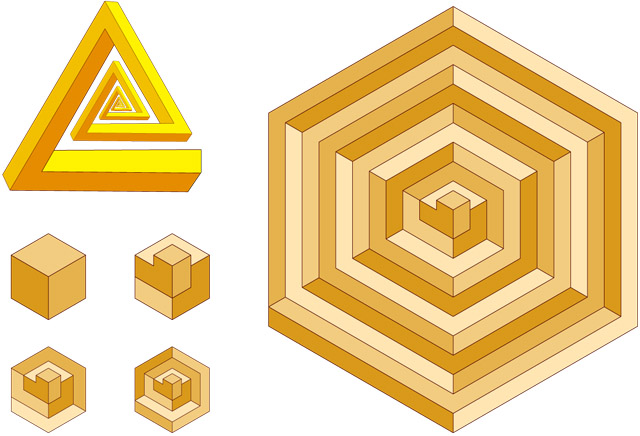Figure 16. A spiral tri-bar and hexagonally bound isometric spirals.